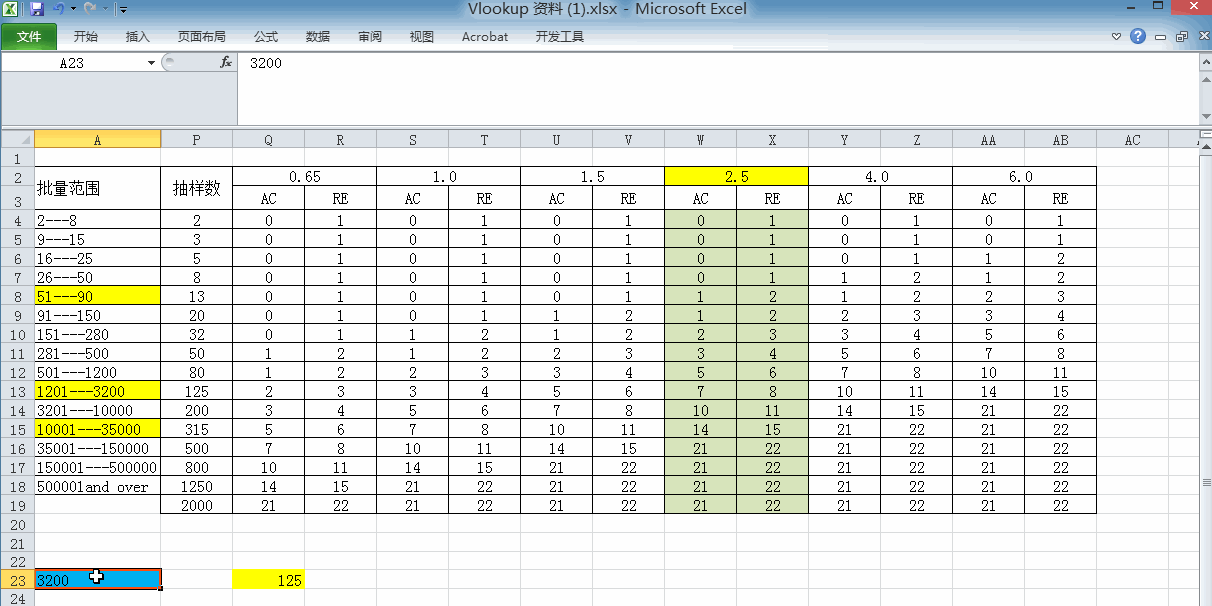# EXCEL分享---IF 函数

二维码 68

=IF(条件,符合条件时的计算方式,不符合条件时的计算方式.)

IF函数的简单运用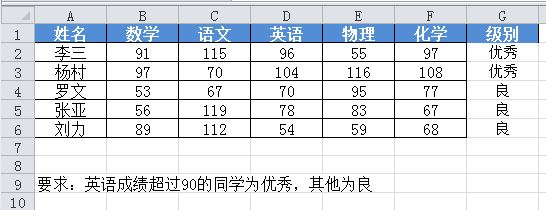IF函数嵌套简单运用（多条件的判断）

AND函数：AND（条件一，条件二.条件三....)      条件一    。。。成立时返回   TURE  否则返回  FALSE表示“且”

OR函数： OR（条件一，条件二.条件三..），只要有一个条件满足就返回TURE  ，所有条件都不满足时返回FALSE表示“或”。

1.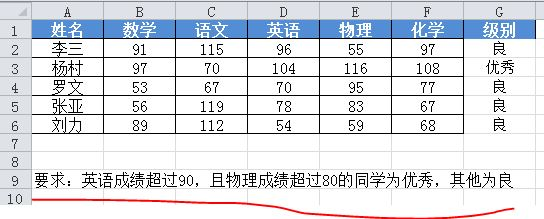2.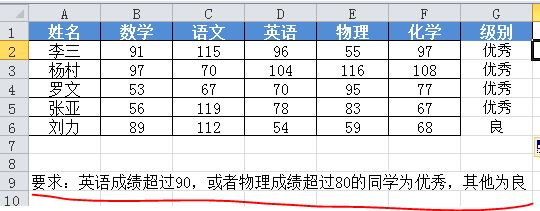公式为：=IF(OR(D2>90,E2>80),"优秀","")IFOR的简单嵌套使用

3.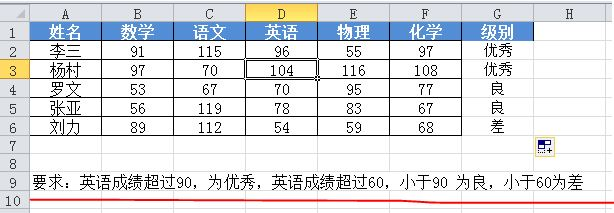IF函数嵌套与Vlookup函数的模糊匹配的对比：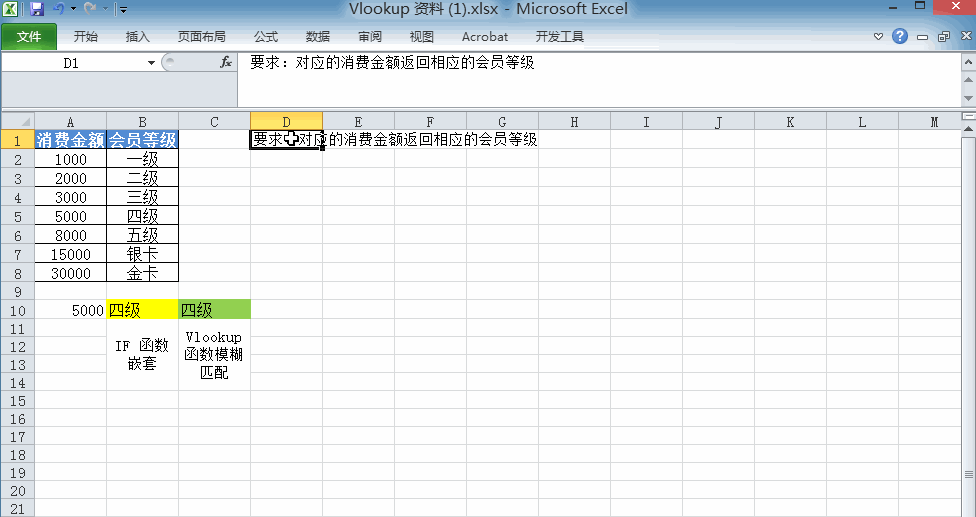IF函数嵌套公式为：=IF(A10>=30000,"金卡",IF(A10>=15000,"银卡",IF(A10>=8000,"五级",IF(A10>=5000,"四级",IF(A10>=3000,"三级",IF(A10>=2000,"二级",IF(A10>=1000,"一级")))))))

Vlookup的模糊匹配公式为：=VLOOKUP(A10,A2:B8,2)上一次提到了Vlookup的模糊匹配：Vlookup函数第四个参数填写1或者True或者不填时，是为模糊查找。意思是，如果找不到精确匹配值，则返回小于要查找值的最大数值。   当输入A10单元格内的数为1500时，没有相对应的会员等级，如果用精确查找的话，返回结果就是错误值了。用模糊查找的话，返回小于要查找的1500这个数的最大数，也就是1000，所以得到的返回值就是会员等级的一级。

IF函数的稍复杂嵌套及扩展：